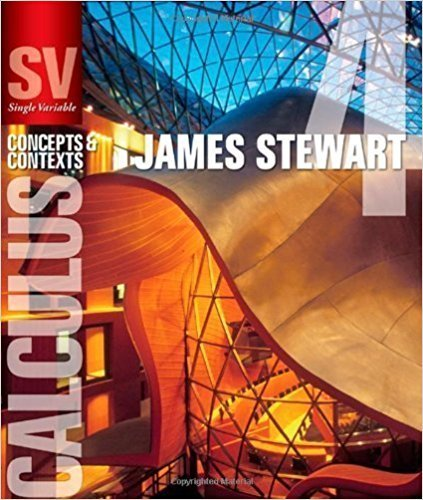×
×

# Solutions for Chapter 6.3: VOLUMES BY CYLINDRICAL SHELLS## Full solutions for Single Variable Calculus: Concepts and Contexts (Stewart's Calculus Series) | 4th Edition

ISBN: 9780495559726Solutions for Chapter 6.3: VOLUMES BY CYLINDRICAL SHELLS

Solutions for Chapter 6.3
4 5 0 341 Reviews
27
2
##### ISBN: 9780495559726

Since 38 problems in chapter 6.3: VOLUMES BY CYLINDRICAL SHELLS have been answered, more than 22355 students have viewed full step-by-step solutions from this chapter. Chapter 6.3: VOLUMES BY CYLINDRICAL SHELLS includes 38 full step-by-step solutions. This textbook survival guide was created for the textbook: Single Variable Calculus: Concepts and Contexts (Stewart's Calculus Series), edition: 4. Single Variable Calculus: Concepts and Contexts (Stewart's Calculus Series) was written by and is associated to the ISBN: 9780495559726. This expansive textbook survival guide covers the following chapters and their solutions.

Key Calculus Terms and definitions covered in this textbook
• Absolute value of a real number

Denoted by |a|, represents the number a or the positive number -a if a < 0.

• Closed interval

An interval that includes its endpoints

• Cosecant

The function y = csc x

• Derivative of ƒ at x a

ƒ'(a) = lim x:a ƒ(x) - ƒ(a) x - a provided the limit exists

• Empty set

A set with no elements

• Equally likely outcomes

Outcomes of an experiment that have the same probability of occurring.

• Horizontal asymptote

The line is a horizontal asymptote of the graph of a function ƒ if lim x:- q ƒ(x) = or lim x: q ƒ(x) = b

• Identity function

The function ƒ(x) = x.

• Inductive step

See Mathematical induction.

• Inverse of a matrix

The inverse of a square matrix A, if it exists, is a matrix B, such that AB = BA = I , where I is an identity matrix.

See Polynomial function in x.

• Quartic regression

A procedure for fitting a quartic function to a set of data.

• Quartile

The first quartile is the median of the lower half of a set of data, the second quartile is the median, and the third quartile is the median of the upper half of the data.

• Quotient identities

tan ?= sin ?cos ?and cot ?= cos ? sin ?

• Range (in statistics)

The difference between the greatest and least values in a data set.

• Richter scale

A logarithmic scale used in measuring the intensity of an earthquake.

• Row echelon form

A matrix in which rows consisting of all 0’s occur only at the bottom of the matrix, the first nonzero entry in any row with nonzero entries is 1, and the leading 1’s move to the right as we move down the rows.

• Secant line of ƒ

A line joining two points of the graph of ƒ.

• Supply curve

p = ƒ(x), where x represents production and p represents price

• Unit circle

A circle with radius 1 centered at the origin.

×